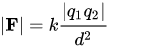Coulombs to electron charge free online conversion

Coulombs (C) to electron charge (e) electric charge conversion calculator and how to convert.

Coulombs to electron charge conversion calculator

Enter the electrical charge in coulombs and press the Convert button:

C
e

Electron charge to coulombs free online conversion »

How to convert coulombs to electron charge

1C = 6.24150975⋅1018e

or

1e = 1.60217646⋅10-19C

Coulombs to electron charge conversion formula

The charge in electron charge Q(e) is equal to the charge in coulombs Q(C) times 6.24150975⋅1018:

Q(e) = Q(C) × 6.24150975⋅1018

Example

Convert 3 coulombs to electron charge:

Q(e) = 3C × 6.24150975⋅1018 = 1.872⋅1019e

Coulomb to electron charge conversion table

Charge (coulomb) Charge (electron charge)
0 C 0 e
1 C 6.24150975⋅1018 e
10 C 6.24150975⋅1019 e
100 C 6.24150975⋅1020 e
1000 C 6.24150975⋅1021 e
10000 C 6.24150975⋅1022 e
100000 C 6.24150975⋅1023 e
1000000 C 6.24150975⋅1024 e

Elementary charge

The elementary charge, usually denoted by e or sometimes qe is the electric charge carried by a single proton or, equivalently, the magnitude of the negative electric charge carried by a single electron, which has charge −1 e. This elementary charge is a fundamental physical constant. To avoid confusion over its sign, e is sometimes called the elementary positive charge.

From the 2019 redefinition of SI base units, which took effect on 20 May 2019, its value is exactly 1.602176634×10−19 C, by definition of the coulomb.

Making the value of the elementary charge exact implies that the value of ε0 (electric constant), which was an exact value before, is now subject to experimental determination: ε0 had an exactly defined value until the 2019 SI redefinition, after which it has become a subject of experimental refinement over time. The SI committees (CGPM, CIPM, etc.) had long considered redefining the SI base units entirely in terms of physical constants so as to remove their dependence on physical artifacts (such as the International Prototype of the Kilogram): for this to work, it was necessary to define fixed values for the physical constants.

Robert A. Millikan's oil drop experiment first measured the magnitude of the elementary charge in 1909.

Coulomb's Law Equation

The quantitative expression for the effect of these three variables on electric force is known as Coulomb's law. Coulomb's law states that the electrical force between two charged objects is directly proportional to the product of the quantity of charge on the objects and inversely proportional to the square of the separation distance between the two objects. In equation form, Coulomb's law can be stated as:where q1 represents the quantity of charge on object 1 (in Coulombs), q2 represents the quantity of charge on object 2 (in Coulombs), and d represents the distance of separation between the two objects (in meters). The symbol k is a proportionality constant known as the Coulomb's law constant. The value of this constant is dependent upon the medium that the charged objects are immersed in. In the case of air, the value is approximately ke ≈ 8.988×109 N⋅m2⋅C−2. If the charged objects are present in water, the value of k can be reduced by as much as a factor of 80. It is worthwhile to point out that the units on k are such that when substituted into the equation the units on charge (Coulombs) and the units on distance (meters) will be canceled, leaving a Newton as the unit of force.

The Coulomb's law equation provides an accurate description of the force between two objects whenever the objects act as point charges. A charged conducting sphere interacts with other charged objects as though all of its charge were located at its center. While the charge is uniformly spread across the surface of the sphere, the center of charge can be considered to be the center of the sphere. The sphere acts as a point charge with its excess charge located at its center. Since Coulomb's law applies to point charges, the distance d in the equation is the distance between the centers of charge for both objects (not the distance between their nearest surfaces).

Currently, we have around 1976 calculators, conversion tables and usefull online tools and software features for students, teaching and teachers, designers and simply for everyone.